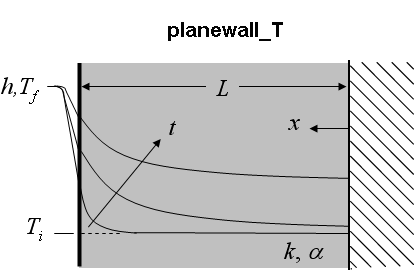Contents - Index

Planewall_TFunction planewall_T(x, time, T_i, T_f, alpha, k, h, L) returns the temperature ([C], [K], [F], or [R]) within a bounded plane wall exposed to convection at one surface (x=L) and adiabatic at the other surface (x=0)

Inputs:
x - distance from adiabatic surface [m] or [ft]
time - time relative to beginning of convection [sec]
T_i - initial temperature of plane wall [C], [K], [F], or [R]
T_f - bulk fluid temperature [C], [K], [F], or [R]
alpha - thermal diffusivity [m^2/s] or [ft^2/hr]
k - thermal conductivity of plane wall [W/m-K] or [Btu/hr-ft-R]
h - heat transfer coefficient [W/m^2-K] or [Btu/hr-ft^2-R]
L -  thickness of plane wall [m] or [ft]

Notes:
Function planewall_T determines the non-dimensional length, Biot and Fourier numbers and calls the function planewall_ND, which determines theta. Theta is then resolved by planewall_T into the temperature.  A separation of variables technique is used to solve for the temperature, as explained in section 3.5.2 of Nellis and Klein.

Example:
\$UnitSystem SI K Pa J
x=0.2 [m]
time=5000 [sec]
T_i=303 [K]
T_f=363 [K]
alpha=8.608e-5 [m^2/s]
k= 233 [W/m-K]
h= 50 [W/m^2-K]
L= 0.5 [m]
T=planewall_T(x, time, T_i, T_f, alpha, k, h, L)

{Solution: T=312.4 [K]}

Transient Conduction Index